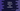# How to subtract two numbers in Python## How to subtract two numbers in Python:

In this post, we will learn how to subtract two numbers in Python with different examples. Subtraction is a basic arithmetic operator and we can subtract one integer or float value from another.

We will learn how to subtract one number from another, how to do chain subtraction with multiple numbers and how to do subtraction by taking the numbers as inputs from the user.

### Subtraction operator:

- is known as the subtraction operator. This operator requires two operands to work. The first one is placed to the left of this operator and it is the number from which we are subtracting. The second one is placed to the right of this operator, which is the value we are subtracting.

Following is the syntax of this operator:

``result_value = first_number - second_number``

It returns the result, i.e. the number we will have by subtracting second_number from first_number.

### Example of subtraction in Python:

Let’s take an example. We will initialize one program with two numbers, subtract one from the another and print the result.

``````first_number = 100
second_number = 40

result = first_number - second_number

print(f'{first_number} - {second_number} = {result}')``````

Here,

• We are finding the subtraction for first_number and second_number.
• first_number is 100 and second_number is 40
• The subtraction result is stored in the result variable.

If you run it, it will print the below output:

``100 - 40 = 60``

### Example of subtracting a bigger number from a smaller number:

Let’s try to subtract a bigger number from a smaller number:

``````first_number = 40
second_number = 100

result = first_number - second_number

print(f'{first_number} - {second_number} = {result}')``````

This example subtracts 100 from 40, i.e. opposite of the previous example. If you run this, it will print:

``40 - 100 = -60``

### Example of subtracting two floating or float numbers in Python:

Let’s try it with two float numbers.

``````first_number = 422.33456
second_number = 122.128898

result = first_number - second_number

print(f'{first_number} - {second_number} = {result}')``````

It will print:

``422.33456 - 122.128898 = 300.205662``

As you can see here, it works similarly with floating numbers as well.

### Example of subtracting two user-given numbers in Python:

Let’s take the numbers as inputs from the users and find the subtraction. This program will ask the user to enter the first and the second number. It will subtract the second number from the first number and print the result.

We can also take the numbers as inputs from the user and print the subtraction result.

``````first_number = int(input('Enter the first number: '))
second_number = int(input('Enter the second number: '))

result = first_number - second_number

print(f'{first_number} - {second_number} = {result}')``````

In this program, we are taking the first and the second number as inputs from the user and it is printing the final result similarly.

It will give output as like below:

``````Enter the first number: 100
Enter the second number: 10
100 - 10 = 90

Enter the first number: 111
Enter the second number: 23
111 - 23 = 88``````